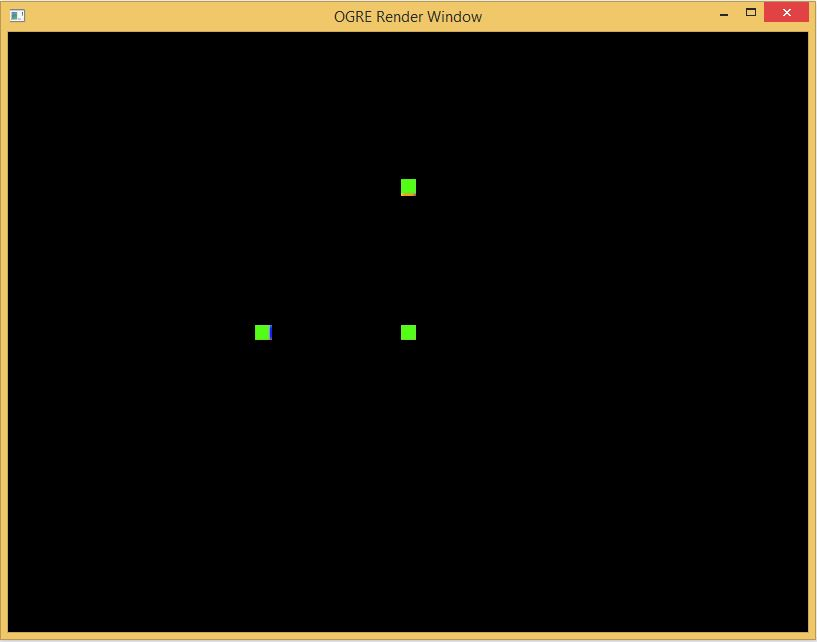Rotation and Translation
Practical Exercises

First, download Translate.zip, and unzip it. Open up the solution Translate, compile, and run. You should see 3 static squares, as follows:Not very exciting, huh? Well, this is supposed to have the blocks orbiting each other. However, the code is not quite correct yet. For this in-class assignment, we will fix the code so that the blocks orbit each other properly.

# Moveable Objects

This project is built around the MovingObject Class (NOTE: The MovingObject class is for demonstration purposes only! MovingObject recreates functionality already in Ogre, so you can see how the math works. Do not use this MovingObject class in your project code!)

## MovingObject data members:

• `MovingObject *mParent`
Parent Pointer If this pointer is null, then the object is in global space. If this parent is non-null, then the rotation and translation of this object is relative to its parent
• `Ogre::Matrix3 mOrientation`
Orientation This is a rotational matrix that gives the orientation (that is, rotation) of the object relative to its parent (or the global orientation, if the object has no parent). Note: We are using Column Vectors for this assignment!
• `Ogre::Vector3 mPosition`
Position This is vector that gives the position of the object relative to its parent (or the global position, if the object has no parent. Note: We are using Row Vectors for this assignment!
• ` Ogre::SceneNode *mObjectSceneNode `
The scene node used to display the object. You will not need to deal with this instance variable for the assignment

## MovingObject Methods

MovingObjects contain the following methods that you do not need to modify

• `Ogre::Matrix3 getOrientation()`
returns the local orientation of the object
• `Ogre::Vector3 getPosition()`
returns the local position of the object
• `void setOrientation(Ogre::Matrix3 orientation)`
sets the local orientation of the object
• `void setPosition(Ogre::Vector3 position)`
sets the local position of the object
• `void translate(Ogre::Vector3 deltaPosition)`
moves the object (in local space)
• `void SetGraphicPosition()`
Set the actual position and rotation of the scene node for this object.

Finally, MovingObjects contain the following methods that you need to modify

• `void yaw(Ogre::Radian theta)`
Rotate the object theta Radians around the y axis. You will need to modify the orientation matrix by creating a new rotation matrix and multiplying it by the old orientation matrix. We will be using column vectors for this assignment, so the basis vectors will be across the columns of the rotational matrix. Currently, this method does nothing, you will need to fill it in.
• `void pitch(Ogre::Radian theta)`
Rotate the object theta Radians around the x axis. You will need to modify the orientation matrix by creating a new rotation matrix and multiplying it by the old orientation matrix. Remember, Ogre3D uses column vectors! Currently, this method does nothing, you will need to fill it in.
• `void roll(Ogre::Radian theta)`
Rotate the object theta Radians around the z axis. You will need to modify the orientation matrix by creating a new rotation matrix and multiplying it by the old orientation matrix. Remember, Ogre3D uses column vectors! Currently, this method does nothing, you will need to fill it in.
• `void GetWorldPositionAndOrientation(Ogre::Vector3 &position, Ogre::Matrix3 &orientation)`
• Return the global position and orientation of the object. Currently, this method returns the local position and orientation, modify it to return the global position and orientation

# Row Vectors vs Column Vectors

Assuming we are using row vectors, then we would rotate a vector v around the z axis using a rotational matrix M as follows:

 x y z

 cos Θ sin Θ 0 -sin Θ cos Θ 0 0 0 1

or, vM. If, However, we are using column vectors, we would rotate the vector v around the z axis as follows:

 cos Θ -sin Θ 0 sin Θ cos Θ 0 0 0 1

 x y z

or Mv. If we wanted to rotate a vector v first by matrix M1, then by M2, then by M3 using row vectors, we would do: vM1M2M3. If, however, we are using column vectors, we would do: M3M2M1v. Ogre3D uses column vectors (and not row vectors!)

# Matrices in Ogre3D

If we wanted to create the following matrix to rotate a column vector by theta degrees around the z axis:

 cos Θ -sin Θ 0 sin Θ cos Θ 0 0 0 1

We could do it in several ways. We could create the matrix directly:

```    Ogre::Radian theta = Ogre::Radian(Ogre::Math::PI / 4);

Ogre::Matrix3 rotate(Ogre::Math::Cos(theta), -Ogre::Math::Sin(theta), 0,
Ogre::Math::Sin(theta), Ogre::Math::Cos(theta),  0,
0,                      0,                       1);
```

Or, we could create the matrix from transformed x/y/z basis vectors, using the FromAxes method. Note that since Ogre uses column vectors, the basis vectors are columns of the created matrix:

```    Ogre::Radian theta = Ogre::Radian(Ogre::Math::PI / 4);

Ogre::Vector3 xBasis = Ogre::Vector3(Ogre::Math::Cos(theta),  Ogre::Math::Sin(theta), 0);
Ogre::Vector3 yBasis = Ogre::Vector3(-Ogre::Math::Sin(theta), Ogre::Math::Cos(theta), 0);
Ogre::Vector3 zBasis = Ogre::Vector3(0,                       0,                      1);
Ogre::Matrix3 rotate;
rotate.FromAxes(xBasis, yBasis, zBasis);
```

Now, if we wanted to use this rotation matrix to rotate a vector v, we would do it as follows:

```    Ogre::Vector3 v;
Ogre::Matrix3 rotate;

// set value for v, create rotation matrix rotate as above
//
v = rotate * v;
```

# What to do

Ready to get to work? Here we go!

• First you should implement the roll / pitch / and yaw methods. Once you have completed these methods and run the program, the cubes should each rotate on a different axis, but should not move around
• Next, implement GetWorldPositionAndOrientation. The cubes should now orbit each other as well as rotating. Also, each cube should rotate relative to its parent. Be sure to look at Changing Coordinate Systems in 2D and Changing Coordinate Systems in 3D
• Make sure your code is correct! The same face of the parent cube should always point to its child!